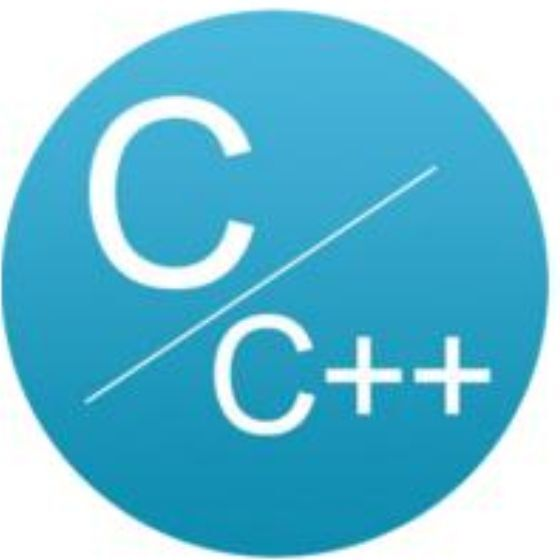# C 语言 /C++ 基本语句编程风格C语言与CPP编程

## 基本语句编程举例

• if 语句

if 语句是 C++/C 语言中最简单、最常用的语句，然而很多编程人员用隐含错误的方式写 if 语句，本文以“与零值比较”为例，进行讨论。

（1）布尔变量与零值比较：不可将布尔变量直接与 TRUE、FALSE 或者 1、0 比较。根据布尔类型的语义，零值为“假”（记为 FALSE），任何非零值都是“真”（记为 TRUE）。TRUE 的值究竟是什么并没有统一的标准，

if（flag）//表示flag为真if（！flag） //表示flag为假

if（flag == TRUE）if(flag == FALSE)if（flag == 1 ）if(flag == 0 )

(2)整型变量与零值比较：应当将整型变量用“==”或“！=”直接与 0 比较。假设整型变量的名字为 value，它与零值比较的标准 if 语句如下：

if(value == 0)if(value != 0)

if(value)if(!value)   //会让人误解value是布尔变量

（3）浮点变量与零值比较：不可以将浮点变量用“==”或“!=”与任何数字比较。

if(x == 0.0)  //隐含错误的比较

if((x >= -EPSINON) && (x <= EPSINON))//其中EPSINON是允许的误差（即精度）。

（4）指针变量与零值比较：应当将指针变量用“==”或“!=”与 NULL 比较。

if(p == NULL)if(p != NULL)   //p与NULL显式比较，强调p是指针变量

if（p == 0）if（p != 0）    //容易让人误解p是整型变量

if(p)if(!p)    //容易让人误解p是布尔变量

（5）对 if 语句的补充说明

if（condition）    return x；return y；

if（condition）{    return x；}else{    return y；}

return（condition ？x：y）；

• 循环语句的效率

C++/C 循环语句中，for 语句使用频率最高，while 语句其次，do 语句很少用。提高循环体效率的基本方法是降低循环体的复杂性。

（1）在多重循环中，如果有可能，应当将最长的循环放在最内层，最短的循环放在最外层，以减少 CPU 跨切循环层的次数。例如下面代码示例 b 的效率就比示例 a 的高。

for（row = 0; row < 100; row++）{   for(col=0;col<5;col++)   {      sum = sum +a[row][col];   }}

for（col = 0; col < 5; col++）{   for(row=0;row<100;row++)   {      sum = sum +a[row][col];   }}

（2）如果循环体内存在逻辑判断，并且循环次数很大，宜将逻辑判断移到循环体的外面。

for（i = 0; i < N; i++）{if(condition)    DoSomthing();else    DoSomthing();}

if (condition){    for (i=0; i<N; i++)        DoSomething();}else{    for (i=0; i<N; i++)      DoSomething();}

(3)for 语句的循环控制变量

for（int x = 0；x < N; x++）{}

for（int x = 0；x <= N-1; x++）{}

switch 语句 switch 是多分支选择语句，而 if 语句只有两个分支可供选择。虽然可以用嵌套的 if 语句来实现多分支选择，但那样的程序冗长难读。switch 语句基本格式：

switch（variable）{    case value1:                  break;    case value2:                  break;        default:		  break;}

• goto 语句

• 由于 goto 语句可以灵活跳转，如果不加限制，它的确会破坏结构化设计风格。

• goto 语句经常带来错误或隐患。它可能跳过了某些对象的构造、变量的初始化、重要的计算等语句，

goto state;String s1, s2; // 被 goto 跳过int sum = 0;   // 被 goto 跳过state:

{ {   {     goto error;   } }}error:

## 小结## 评论